Principal analytic fibration

A locally trivial analytic fibration on the fibres of which the structure Lie group acts simply transitively and analytically. In other words, a principal analytic fibration is a quadruplewhereandare analytic spaces (cf. Analytic space) over a field;is an analytic mapping;is a Lie group overanalytically acting onfrom the right; and for each element of the basethere exist a neighbourhoodand an analytic isomorphism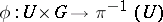such thatEach analytic vector bundle (cf. Vector bundle, analytic)with-dimensional fibre determines a principal analytic fibration with baseand group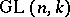, whose fibre over a point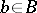is the variety of all bases of the fibre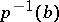. This is a special case of a one-to-one correspondence between analytic fibrations with given fibre and structure groupand the principal analytic fibrations associated with them. Other examples of principal analytic fibrations include the fibration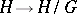whose fibres are the left cosets of the Lie groupby a Lie subgroupof it, and an analytic covering (here, the group of the covering is the structure group).

A principal analytic fibration can be defined by an open covering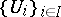of its baseand transition functions, i.e. analytic mappingswhich satisfy the conditions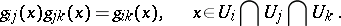The transition functions form a one-dimensional cocycle with values in the sheaf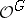of germs of analytic mappings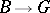. This yields a one-to-one correspondence between the set of principal analytic fibrations with baseand groupand the set of cohomology spaces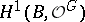. The classification of the principal analytic fibrations with baseand structure group(in the case of a non-Abelian group) is a problem which has so far been solved in a number of cases only (cf. Deformation of an analytic structure, for the corresponding local moduli problem). The connection between this classification and the classification of principal topological fibrations is expressed by the natural mappingwhere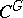is the sheaf of germs of continuous mappings.

Let, and letbe a reduced Stein space; the mappingis then bijective, and the classification problem is reduced to a problem in homotopy theory . In particular, any principal analytic fibration with a non-compact Riemann surface as base and with a connected structure group is trivial. The result of  was in fact obtained for the broader class of-principal fibrations, in the definition of which the groupis replaced by some locally trivial analytic fibration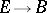on complex Lie groups, acting fibre-wise on. This result has been generalized to the case when the structure group is a complex Banach Lie group . If, on the other hand,is a compact Riemann surface, thenis surjective and not injective. In the general caseneed not be either surjective nor injective. Sufficient cohomological conditions forto be injective or surjective are known  for a connected solvable group.

The case whenis a compact Riemann surface of genusandis a connected reductive algebraic group has been also studied. See  for the corresponding classification for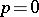; see  for the corresponding classification forwhen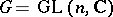. In the case,(cf. Vector bundle, algebraic) an important role is played by the concept of a stable vector bundle. This concept has been generalized  to the case of an arbitrary connected reductive group. The set of all stable principal fibrations of a given topological type on a compact Riemann surface has the natural structure of a connected normal analytic space. A partial classification of vector bundles over the complex projective space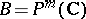and over algebraic surfaces has also been obtained.

See Real-analytic space for real principal analytic fibrations (the case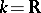).